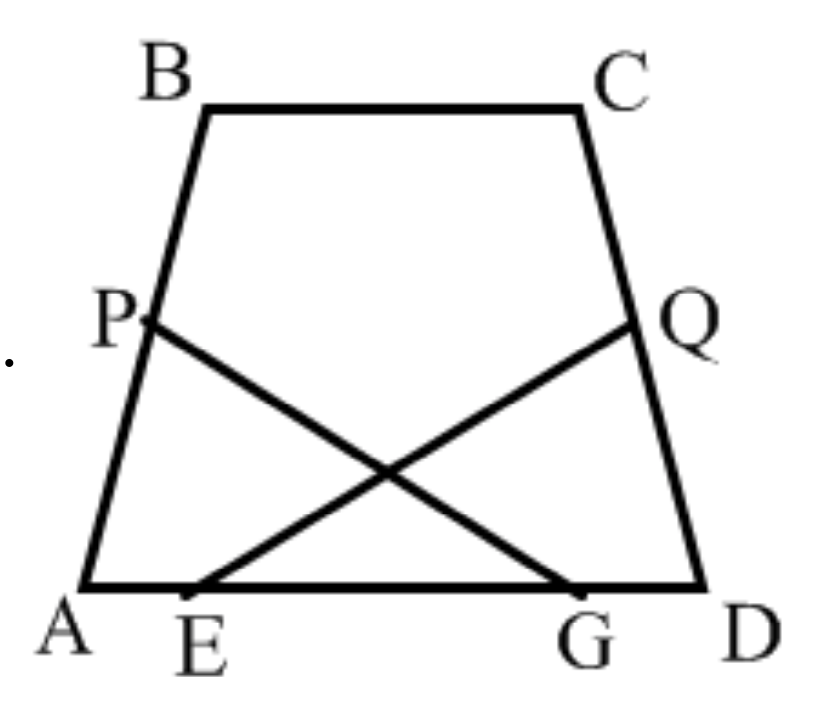### Prove: △AGP ≅ △DEQClick or touch to draw on the diagram
Substitution
Algebra
Perpendicular lines form right angles
Substitution
ASA (Angle-Side-Angle)
Definition
$$PA = \frac{1}{2}BA$$ and $$QD = \frac{1}{2}CD$$
$$PA = \frac{1}{2}BA$$ and $$QD = \frac{1}{2}BA$$
$$\angle APG = \angle EQD = 90^\circ$$